# Decision Trees v/s Random Forests

## Introduction

Do you ever feel like a person lost in a random forest when it comes to deciding whether to use a decision tree or a random forest? Pun intended!  How are decision trees different from random forests? To answer this question, let us dive into the topic: decision trees v/s random forests! But first, we have to know what a decision tree is and what a random forest is!

decision tree comes under supervised machine learning techniques. As the name implies, it uses a tree-like flowchart to display the predictions resulting from a sequence of feature-based splits. A random forest is also a supervised machine learning technique that uses decision tree algorithms to build it. It uses ensemble learning, a method for solving complicated problems by combining several classifiers.

Decision trees v/s random forests, what should you choose to solve the regression and classification problems!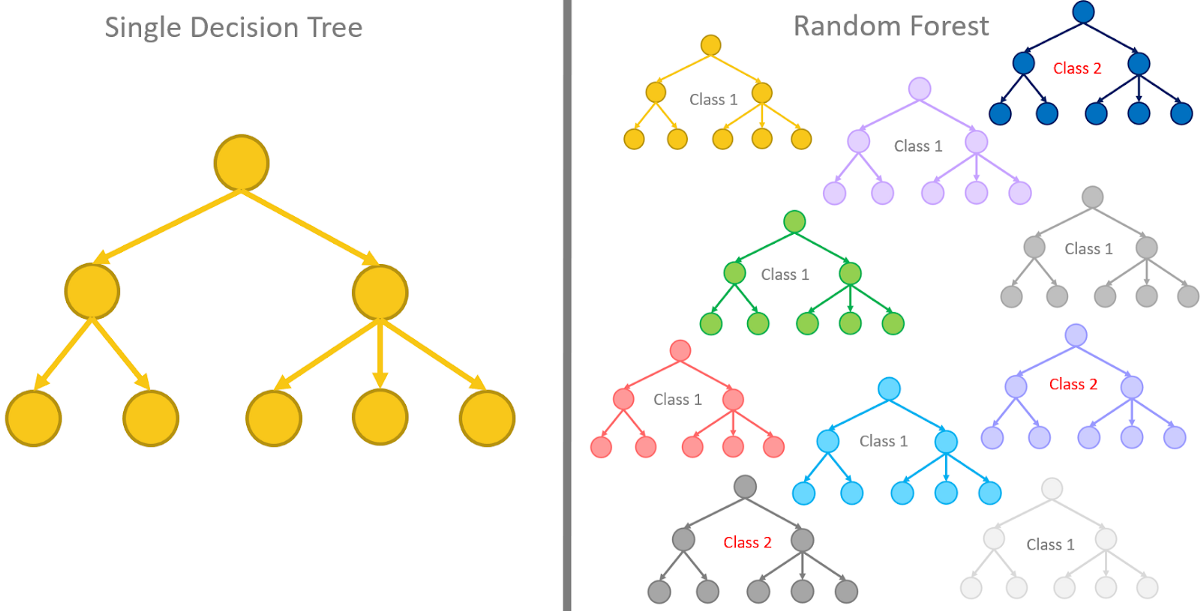Source

The advantages of decision trees are as follows:

- They are simple and easy to understand: Split the data and make nodes of the tree after calculating the Gini index or information gain for each feature until you take all the features into account and have reached the leaf node where the final decision is made.

- It is easy to visualize it: It is just a tree with a root node branching out to other nodes and finally to the leaf node, after all!

- They are fast and can handle both categorical and numerical data!

The disadvantages of decision trees are as follows:

- A decision tree is prone to overfitting. That is, a decision tree may work well on the training data but may not make a good prediction on testing data!

The code below demonstrates an example of overfitting using the decision tree classifier from the Sklearn library on the iris dataset.

``````#imports
import pandas as pd
import numpy as np
from sklearn.model_selection import train_test_split
from sklearn.tree import DecisionTreeClassifier
from sklearn.ensemble import RandomForestClassifier
from sklearn.metrics import confusion_matrix
from sklearn.metrics import f1_score
import matplotlib.pyplot as plt
import seaborn as sns
#forming dataframe with it
data_df=pd.DataFrame(data.data,columns= ['sepal length (cm)',
'sepal width (cm)',
'petal length (cm)',
'petal width (cm)'])
#features
data_df``````
``````#target
target_df=pd.DataFrame(data.target,columns= ["name"])
target_df``````

``````x=data.data
y=data.target
#splitting iris data to training and testing
x_train,x_test,y_train,y_test=train_test_split(x,y,random_state=50,test_size=0.25)``````

Make a function that builds a decision tree, prints the f1 scores and displays the confusion matrix. The F1 score is nothing but the harmonic mean of Precision and Recall. In this case, we will use the micro F1 score.

Source

``````def decision_tree_func(x_train,x_test,y_train,y_test):
decision_tree_clf=DecisionTreeClassifier(random_state=42)
#fitting the data into the decision tree classifier
decision_tree_clf.fit(x_train,y_train)
#predicting y for both testing and training data
y_pred=decision_tree_clf.predict(x_test)
y_train_pred=decision_tree_clf.predict(x_train)
print("DECISION TREE:")
print('Training Set Evaluation F1-Score:',f1_score(y_train,y_train_pred, average='micro'))
print('Testing Set Evaluation F1-Score:',f1_score(y_test,y_pred,average='micro'))
#CONFUSION MATRIX
ax = sns.heatmap(confusion_matrix(y_train, y_train_pred), annot=True, cmap='Blues')
ax.set_title('Confusion Matrix for training dataset\n\n');
ax.set_xlabel('\nPredicted Values')
ax.set_ylabel('Actual Values ');
## Display the visualization of the Confusion Matrix for training data set
print(plt.show())
ax2 = sns.heatmap(confusion_matrix(y_test, y_pred), annot=True, cmap='Blues')
ax2.set_title('Confusion Matrix for testing dataset\n\n');
ax2.set_xlabel('\nPredicted Values')
ax2.set_ylabel('Actual Values ');
## Display the visualization of the Confusion Matrix for testing data set
print(plt.show())``````

Now, the moment of truth:

``decision_tree_func(x_train,x_test,y_train,y_test)``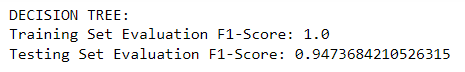You can see that the f1 score we got is 1, which clearly shows that the model overfitted on training data.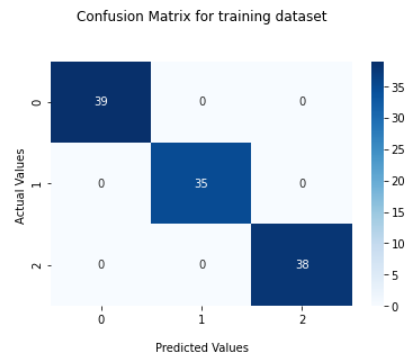In the confusion matrix above, for training data, there are 0 false positives and 0 false negatives for every category (0,1,2). Thus we can conclude that all target values in the training dataset were predicted correctly.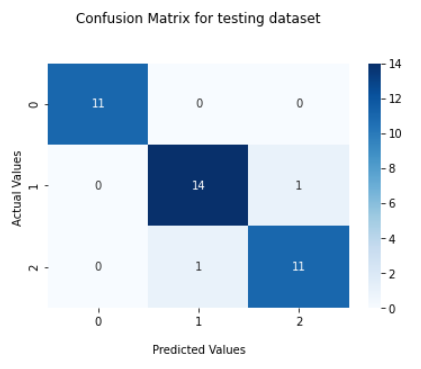The confusion matrix for the testing data shows that:

• All the 11 values belonging to “category 0” were predicted correctly
• 14 values of “category 1” were predicted correctly, while 1 value was given the wrong “category 2.”
• Eleven values of “category 2” were predicted correctly, while one was given the wrong “category 2.”

This model is also performing well on testing data because iris is a small data set with almost no outliers. However, you will see how lousy decision trees can be when datasets have outliers and a vast number of features in the other section.

- Pruning is a cumbersome process. Pruning is a data compression technique used in machine learning and search algorithms to minimize the size of decision trees by deleting non-critical and redundant elements of the tree. Pruning minimizes the final classifier's complexity, which increases predicted accuracy by reducing overfitting.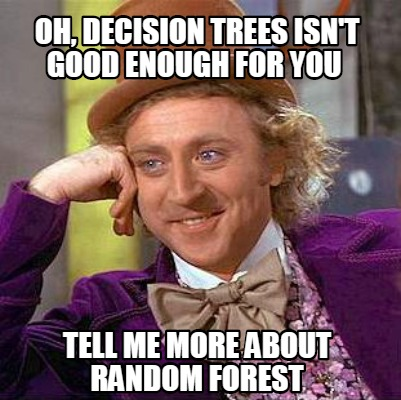Source

As discussed before, random forests are a collection of many decision trees. It is a supervised machine learning method that is a development of the original bagging algorithm and is based on ensemble learning. There are many advantages of a random forest. However, all boils down to a few things: The random forest algorithm is more accurate at predicting outcomes than the decision tree method and can run the trees in parallel ways. It can handle large datasets efficiently.

The disadvantages of random forests are: Needs additional computing resources are required. And when compared to a decision tree algorithm, it takes longer.

Picture of a random forest (I am sure you got the reference)Source

## Why use random forests?

We learned from the earlier section that Random forests are an enhancement of the original Bagging method which is an ensemble technique.

Bootstrap sampling (bagging) is used to create numerous different models from a single training dataset. Random forests add a random variation to the bagging technique to increase model variety. Random forests are based on creating many decision trees and combining them to produce a more accurate result.

Source

Random forests force the trees to divide on only a subset of its predictors throughout the growing phase. Because each tree is generated on a separate random subset of data, all decision trees that make up a random forest are unique. It is more accurate than a single decision tree because it minimizes overfitting.

## Decision Trees and Radom Forests: A quick comparison!

Decision Trees v/s Random Forests

### Decision trees v/s random forests: In code!

Let us see the difference in the performance of decision trees and random forests in code. Let’s work on the infamous Titanic dataset. The Titanic dataset has testing data with no column for people who survived. So, we will work on the training data of the titanic dataset.

Note: Continue from the previous code.

``````def random_forest_func(x_train,x_test,y_train,y_test):
random_forest_clf=RandomForestClassifier(random_state=42)
#fitting the data into the random forest classifier
random_forest_clf.fit(x_train,y_train)
#predicting y for both testing and training data
y_pred=random_forest_clf.predict(x_test)
y_train_pred=random_forest_clf.predict(x_train)
print("RANDOM FOREST:")
print('Training Set Evaluation F1-Score:',f1_score(y_train,y_train_pred, average='micro'))
print('Testing Set Evaluation F1-Score:',f1_score(y_test,y_pred,average='micro'))
#CONFUSION MATRIX
ax = sns.heatmap(confusion_matrix(y_train, y_train_pred), annot=True, cmap='Blues')
ax.set_title('Confusion Matrix for testing dataset\n\n');
ax.set_xlabel('\nPredicted Values')
ax.set_ylabel('Actual Values ');
## Display the visualization of the Confusion Matrix for training data set
print(plt.show())
ax2 = sns.heatmap(confusion_matrix(y_test, y_pred), annot=True, cmap='Blues')
ax2.set_title('Confusion Matrix for testing dataset\n\n');
ax2.set_xlabel('\nPredicted Values')
ax2.set_ylabel('Actual Values ');
## Display the visualization of the Confusion Matrix for testing data set
print(plt.show())``````

Let us download the training data CSV file for the Titanic dataset. Note that we are only going to work on the training dataset of the Titanic dataset.

``````#reading csv file and making DataFrame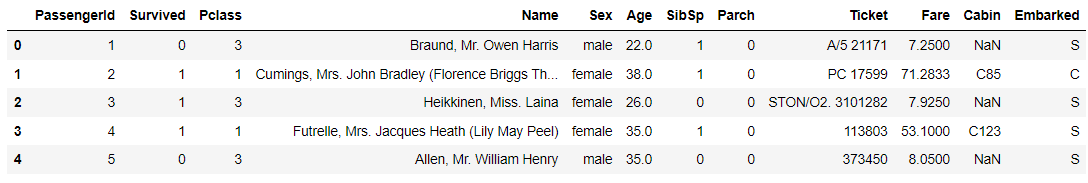Doing the best preprocessing of data is not the priority.

``````#dropping rows with Nan
titanic_df=titanic.dropna(axis = 0)
#dropping columns we won't use in the classifiers
titanic_df=titanic_df.drop(["PassengerId","Pclass","Name","Ticket","Fare","Cabin","Embarked"], axis = 1)
#Replacing Female with 1 and Male with 0 in the column for sex
def female_or_male(x):
if(str(x)=="female"):
return 1
else:
return 0
titanic_df["Sex"]=titanic_df["Sex"].apply(lambda x:female_or_male(x))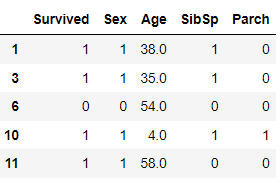``````#Dividing the data into features and target array
y_titanic=titanic_df["Survived"].to_numpy()
titanic_df_copy=titanic_df.copy()
del titanic_df_copy["Survived"]
x_titanic=titanic_df_copy.to_numpy()
#Split the data into training and testing data
x_train_titanic,x_test_titanic,y_train_titanic,y_test_titanic=train_test_split(x_titanic,y_titanic,random_state=50,test_size=0.25)``````

Now for the moment, you’ve all been waiting for: Decision trees v/s random forests code!

``````#Calling the decision_tree_func function and passing the training and testing data
decision_tree_func(x_train_titanic,x_test_titanic,y_train_titanic,y_test_titanic)
print("\n")
#Calling the random_forest_func function and passing the training and testing data
random_forest_func(x_train_titanic,x_test_titanic,y_train_titanic,y_test_titanic)``````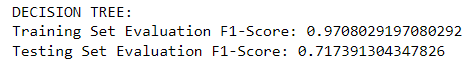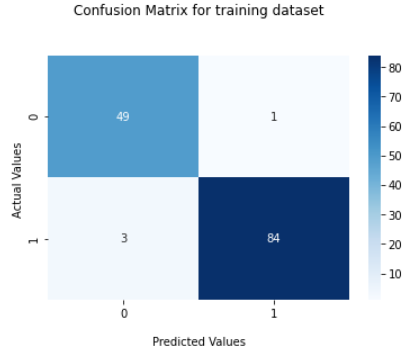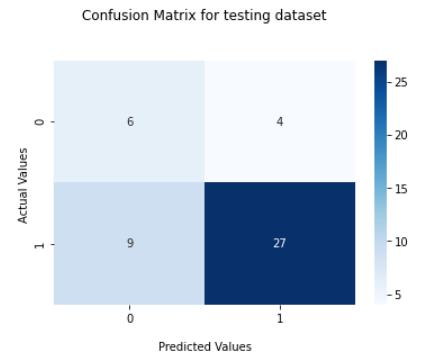Let us look at the output for the random forest function: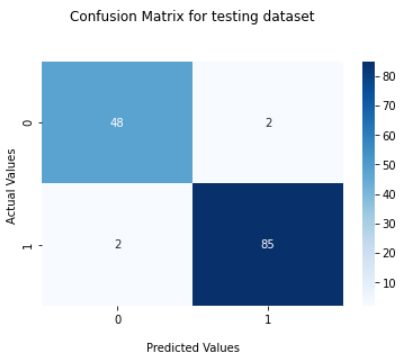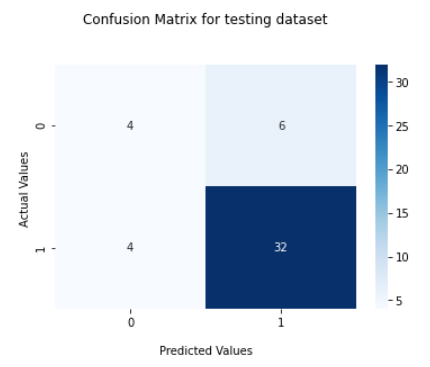We see that the random forest has a better F1 score than the decision tree and hence performs better. You can also compare the confusion matrices for better clarity.

1. When should you utilize a decision tree?
Answer. It would help if you used a decision tree to make your model easy to understand and not parametric. Use it when you don't want to be bothered with feature selection, regularisation, or multi-collinearity. You can overfit the tree and develop a model if you're confident that the validation or test data set will be a subset of the training data set or nearly overlapping rather than unexpected.

2. When should you utilize a random forest?
Answer. It would be best to use random forest when you don't care about the model's interpretation but want it to be more accurate. Because random forest reduces the variance of error rather than the bias, decision trees may be more accurate than random forests on a given training data set. On the other hand, random forest always wins in terms of accuracy on an unexpected validation data set.

3. What are some of the applications of Random forest?
Answer. We can use it for a variety of purposes, including:
• Banking
A random forest is used in banking to determine a loan applicant's creditworthiness. It assists lending organizations in making an informed judgment on whether or not to grant the loan to the consumer. Banks often use the random forest technique to detect fraudsters.
• Health-care services
Doctors use random forest algorithms to diagnose patients. Patients' historical medical records are used to diagnose them and determine the correct dosage for the patients. Previous medical data is evaluated.
• The stock exchange
Financial gurus use it to identify potential stock markets. They can also recognize stock activity using it.
• E-commerce
E-commerce merchants can forecast customer preferences based on prior consumption behavior using rain forest algorithms.

## Key Takeaways

In this article, we dived into the topic of decision trees v/s random forests. We saw the similarities and differences between decision trees and random forests. We saw when and why to use random forests over decision trees and how they solve the problem of overfitting. We noticed that random forest performs better than decision tree through code. If you want to learn more about them, check out our industry-oriented machine learning course curated by our faculty from Stanford University and Industry experts.